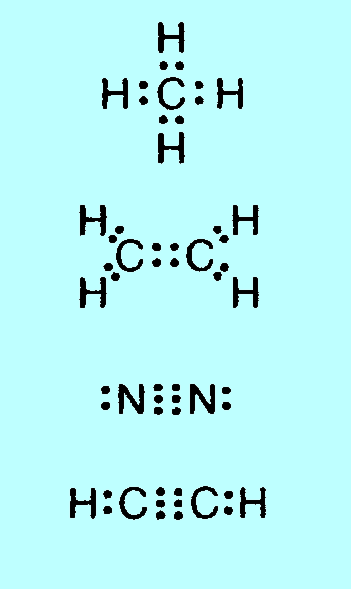Errors in Conquering Chemistry  Preliminary Course 4th edition

If you discover any other errors, please let me know on rolandh@tpg.com.au.

 p. 90 right-hand column of          table, 6th row: change 1.14 to 1.13 (should read 1.5 to 1.13) next row (starting assess          separation ...): change 1.13 to 1.14 p. 113 equation (4.2): Should be just Cu2S  (not 2Cu2S) p. 127 question 23 d: Change mercury to silver p. 157 question 2 (3rd line): after ...82.5% indium add and that oxygen had an atomic weight of 16 p. 316 question 6 The test described does not allow you to determine whether the shiny grey substance is an element or a compound; all it tells you is that it is a pure substance (definite melting point). There are many compounds that are quite stable up to 327oC, so the lack of decomposition does not prove that it is an element. (This was a real boo-boo on my part; sorry.) p. 321 question 40: 8.2 t (not 8.3 t) p. 321 question 41: 0.36 t (not 0.26 t) p. 322 question 13 a: After RbNO3 insert Cs2SO4

The following errors occur in the first printing of the fourth edition (2004). They have been corrected in subsequent printings. You can tell which printing your copy of the book belongs to by looking near the top of page iv (opposite the table of contents); if it has a reprint date later than 2004 the errors below will have been corrected.

 p. 29 line 5: separates (one t only) p. 40 caption to Fig 4.8: nanometres (n not m) p. 50 last line of text: CaF2  (subscript 2) p.52 4th line of example 3: Cl2  (subscript 2) p. 59 exercise 31: should be an asterisk in front of the 31   (i.e. no answer given) p. 63 exercise 33: should be an asterisk in front of the 33   (i.e. no answer given) p. 66 question 46: The headings for the 4th and 5th columns should be set out as:  Electrical conductance  Pure                 In aq. soln That is, electrical conductance refers to both columns p. 76 4th line from bottom: Mg2+   (superscript 2+) p. 77 Table 3.2, 4th row: delete H– p. 90 right-hand column of          table, 6th row: change 1.12 to 1.13 and 1.14 to 1.15 next row: change 1.13 to 1.14 next row: change 1.19 to 1.20 bottom row: change 1.20, 1.21 to 1.21, 1.22 p. 91 1st row after heading: change 1.22 to 1.23 next row: delete 1.23 p.102 bottom row of table: 'for both balance is roughly equal amounts of silver and gold' should be in the second (composition) column, under '37.5% gold' p. 113 equation (4.2) Should be just Cu2S  (not 2Cu2S) p. 139 in the yellow-green           shading: AwByCz should be AwByCz  (w, y, z subscripts) both in the 1st line and in the bottom line of Equation 5.3 p. 143 5th line (in the            equation): change 2H2(l) to 2H2O(l)   (i.e. insert O 8th, 9th, 10th, 11th lines: change O2 to CO2   (four times) p. 189 5th line after Fig 7.3: change 6.4 to 6.5 p. 191 Fig 7.6, in the text at          the top, after Od–: insert 'or Nd–' p. 199 Table 7.5 4th blue             row: line up   '· a few soluble'  with  '· starch, glycogen, enzymes' p. 205 Table 8.1 bottom line: '(except Groups 1, 2 and NH4+)' should line up with  '· sulfides' p. 214 5th line Example 6 c: 4th line     106  not  106 p. 219 Example 11 4th line: in the chemical equation ´ should be + p. 220 4th line: delete –1  (i.e. g/mol) p. 252 set of dot and ?        structures in the middle       of the page: There are 4 structures missing on the left-hand side of this diagram, the all-black-dot ones:p. 264 5th line: Table 9.9   (not Figure 9.9) p. 265 5th line: insert 'compound' after 'straight-chain'  (a straight-chain compound with five carbon?) p. 267 Figure 9.11, bottom           left-hand shaded bit: first CH2 should be CH3 p. 299 7th line: 103 to 106  (not 103 to 106 ) p. 314 right-hand column      Ans 14 3rd line: Ca not Sr Ans 15 1st line: underline 3 and 6   (B 2, 3; O 2. 6  ) 2nd line: underline last 2    (Ca 2, 8, 8, 2  ) 3rd line: underline 2 in Mn and 6 in Se  (Mn 2, 8, 13, 2; Se 2, 8, 18, 6   ) 4th line: underline final 1   (Rb 2, 8, 18, 8, 1   ) p. 315 Ans 11 d: delete (h)  (d CaH2 ) Ans 18 a: dinitrogen monoxide (not dinitrogen oxide) p. 327 Ans 14 b: Mg(NO3)2.6H2O  (not  MgSO4.6H2O) p. 328 Ans 16 a: change 2.0 to 3.2   (3.2´ 10–3 g  ) Ans 17: at end of line: insert C8H16 p. 334 Ans 8: Figure 10.1(b)    (not 10.21(b)  ) p. 341 in index insert 'solutions' after 'apparatus for measuring'

Errors in Conquering Chemistry Preliminary Course Teacher Resource CD

 Module 2 Worksheet 13 (with answers)                 Question 11 b: %O = (9 x 16) / (2 x 27 + ... = 61.5%   (9 missing, answer wrong) Question 12 a: %O = (4 x 16 / 154.8) x 100 = 41.3%  (4 missing, answer correct) Module 2 Worksheet 14 (with answers)                  Question 9 b: the chemical equation should end with + H2O  (not H2)